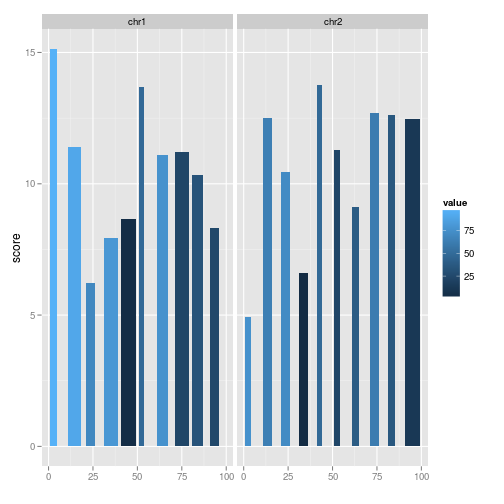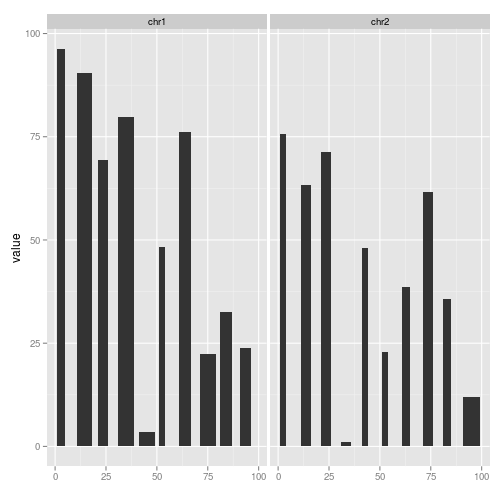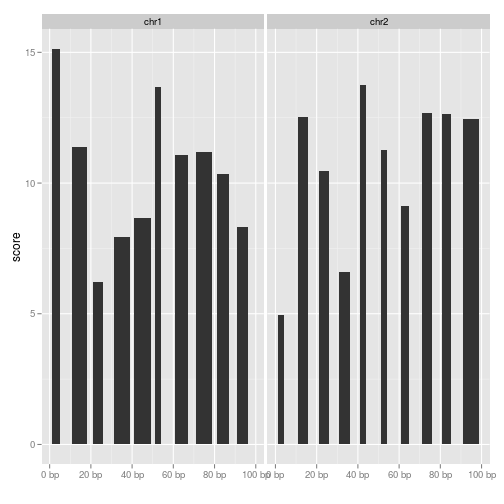geom_bar {ggbio} R Documentation

## Segment geoms for GRanges object

### Description

Show interval data as vertical bar, width equals to interval width and use 'score' or specified 'y' as y scale.

### Usage

```##  for data.frame
## S4 method for signature 'data.frame'
geom_bar(data, ...)

## S4 method for signature 'GRanges'
geom_bar(data,..., xlab, ylab, main)

```

### Arguments

 `data` A `GRanges` or `data.frame` object. `...` Extra parameters such as aes() or `color, size` passed. `xlab` Label for x `ylab` Label for y `main` Title for plot.

### Details

Useful for showing bed like files, when imported as GRanges, have a extra 'score' column, use it as default y, you could also specify y by using aes(y = ).

A 'Layer'.

### Examples

``````## load
library(GenomicRanges)
## simul
set.seed(123)
gr.b <- GRanges(seqnames = "chr1", IRanges(start = seq(1, 100, by = 10),
width = sample(4:9, size = 10, replace = TRUE)),
score = rnorm(10, 10, 3), value = runif(10, 1, 100))
gr.b2 <- GRanges(seqnames = "chr2", IRanges(start = seq(1, 100, by = 10),
width = sample(4:9, size = 10, replace = TRUE)),
score = rnorm(10, 10, 3), value = runif(10, 1, 100))
gr.b <- c(gr.b, gr.b2)
``````
``````## Warning: Each of the 2 combined objects has sequence levels not in the
## other: - in 'x': chr1 - in 'y': chr2 Make sure to always combine/compare
## objects based on the same reference genome (use suppressWarnings() to
## suppress this warning).
``````
``````## default use score as y
## bar
ggplot(gr.b) + geom_bar(aes(fill = value))
``````
``````## use score as y by default
````````````## or
ggplot() + geom_bar(gr.b, aes(fill = value))
``````
``````## use score as y by default
````````````ggplot(gr.b) + geom_bar(aes(y = value))
````````````## equal to
autoplot(gr.b, geom = "bar")
``````
``````## use score as y by default
``````[Package ggbio version 1.5.20 ]